Get instant live expert help with Excel or Google Sheets“My Excelchat expert helped me in less than 20 minutes, saving me what would have been 5 hours of work!”

#### Post your problem and you’ll get Expert help in seconds.

Your message must be at least 40 characters
Our professional Expert are available now. Your privacy is guaranteed.

# Guide to Creating a Timesheet in Excel

As such, there is no specific formula of creating all the elements of timesheet at once. It’s a step by step process of putting few different formulas and keys in Excel. And FInal Result can be seen below: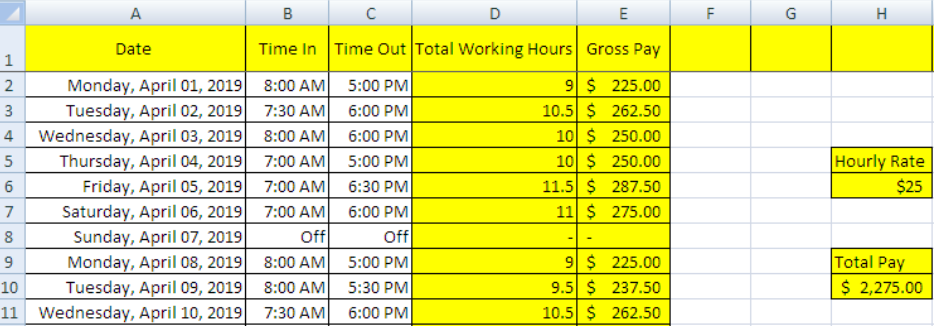Figure 1 – Final Result

Step 1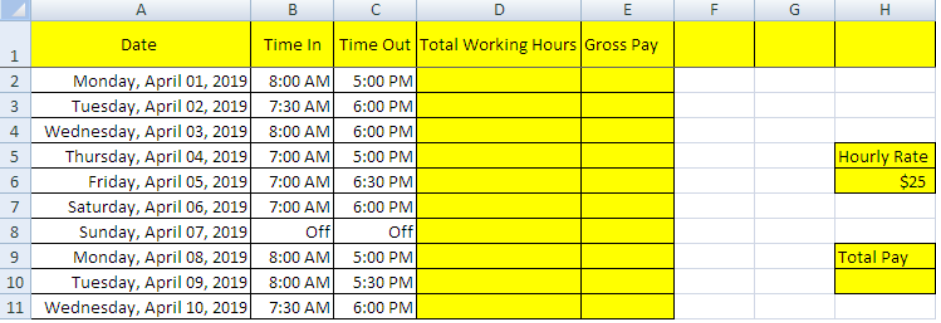Figure 2 – Setting up data

This is an example of an individual that works in a company and gets paid on hourly basis.

• Categorize the cells in the first row according to your requirements.
• Click on column A, select “format cells”, click on “date” and choose the format of date.
• Write date in A2 and drag it to A11. The date for the next 9 days will automatically appear.
• Time in and Time Out is filled on daily basis by the individual.

Step 2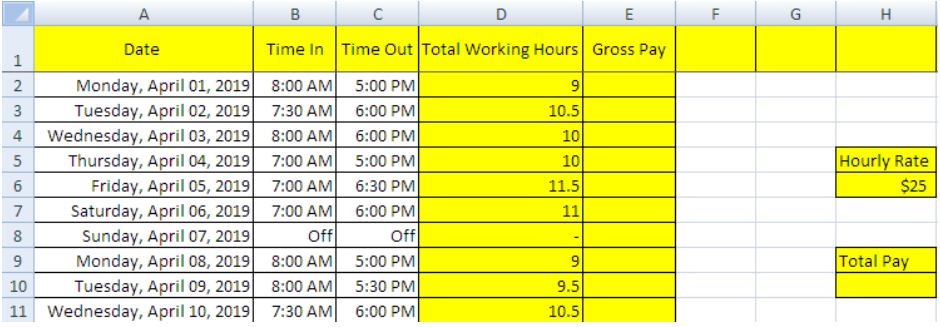Figure 3 – Calculate “Total number of working hours”

In this step, we will find out the “Total Number of working Hours” by using a formula.

Formula: `=(time out-time in)*24`

Or

`=(C2-B2)* 24 in this case`

• Calculate the total working hours by entering the formula `=(C2-B2)*24` in cell D2.
• Select cell D2 and copy the formula, then select column D and choose “formulas” from “pasting options”.
• Excel will automatically calculate the working hours for the rest of the days.

Step 3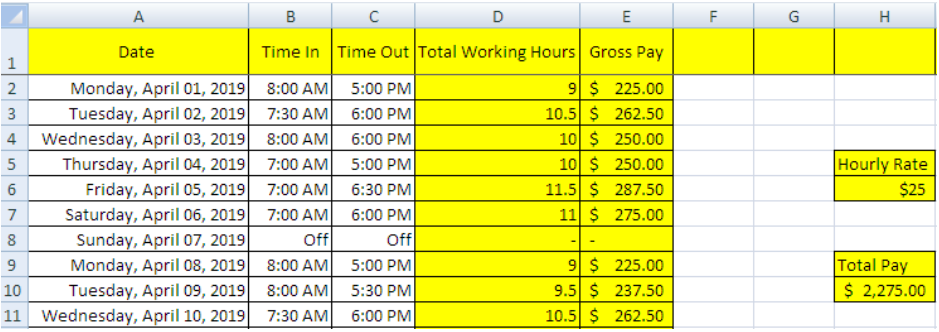Figure 4 – Calculate “Gross Pay”

In step no.3, we are going to find out “Gross Pay” with the help of another simple formula.

Formula: `=Hourly rate*Working hours`

Or

` =H6*D2` in this case

• Write formula `=H6*D2 `in the cell E2 to calculate gross pay.
• Lock the formula by clicking “F4” and press “Enter”.
• Copy the formula from cell E2 and then click column E and select pasting formulas.

Step 4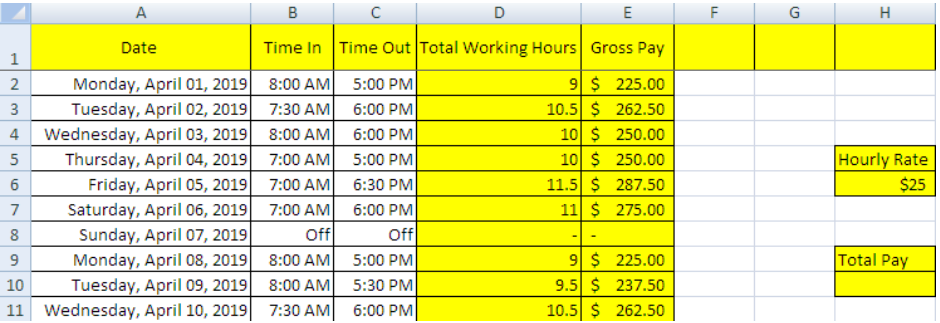Figure 5 – Final result

The final step of the worksheet is to calculate the payment of the worker for 10 days.

Formula: `=SUM(Gross Pay Column)`

Or

` =SUM(E:E)`

• Select cell H10.
• Write `=SUM(E:E)` and press Enter.
• Instead of writing E:E, you can also simply click on column E to get the same result.

## Notes:

• We can continue with the same timesheet for the 11th working day or for the whole month.
• Excel will automatically calculate the gross pay and total pay on entering data every day.
• While entering formulas, if we counter with #VALUE! Or #####, we can click the text and “delete” and re-write the text of our choice.

Most of the time, the problem you will need to solve will be more complex than a simple application of a formula or function. If you want to save hours of research and frustration, try our live Excelchat service! Our Excel Experts are available 24/7 to answer any Excel question you may have. We guarantee a connection within 30 seconds and a customized solution within 20 minutes.

Solution examplesI need a formula that has these 2 rules. If letter was rec'd between 1st and 15th = 1st of the month following letter rec'd date. If letter was rec'd between 16thst and end of the month= 1st of the 2nd month following letter rec'd date.
Solved by V. F. in 20 minsI need a column to total (Hours)(85) + (Travel)(.55) per line
Solved by F. Q. in 20 minsTrying to figure out what is wrong with my formula in Oct-ZD tab in column AY and BG.
Solved by O. Q. in 8 minsSo I have used vlookup to find the price of a product on a different sheet and then I have tried to times that by a quantity. e.g. =vlookup(d4,table35,2,false)*e4 This is the formula I typed in which worked for another list I did but for some reason it's not working now even though everything is practically the same, apart from the contents. Could you help?
Solved by B. U. in 60 minsI have a column containing date and time data together and i need to isolate the time data. When I tried to use text to column, it changed all the times to AM. There is an extremely large amount of data - over 550,000 rows so manually changing it won't work. The file is too large to attach.
Solved by S. E. in 40 mins